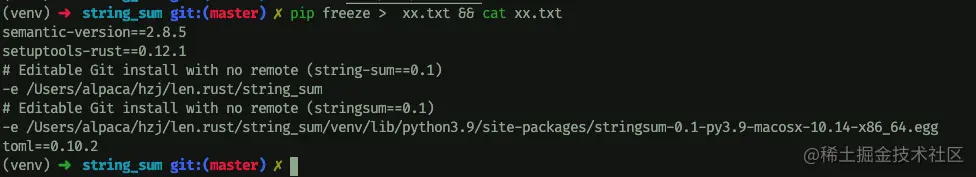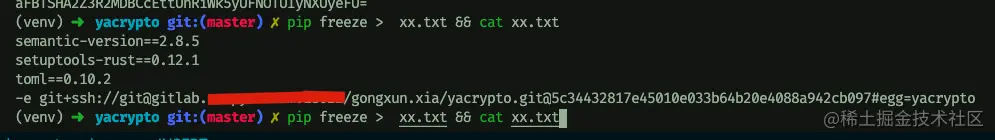# Practical experience sharing: use pyo3 to build your Python module

2022-01-29 17:26:22

PyO3 It is mainly used to create native files Python Expansion module for .PyO3 Also support from Rust Binary run Python Code and interact with it , Can achieve rust And Python Code coexistence . On some modules with high performance requirements , Consider using PyO3 Build corresponding functional modules .PyO3 The separation of functions , Don't worry too much about the coupling between modules , And can have a certain improvement in speed .

github Address : github.com/PyO3/pyo3

The version is as follows ：

• Python 3.6+

• Rust 1.41+

Next we go through a small demo Learn from PyO3 Compile module to Python The whole process normally used in .

``````cargo new --lib string-sum
Copy code ``````

## Create project

``````# lib.rs
[package]
name = "string-sum"
version = "0.1.0"
edition = "2018"

[lib]
name = "string_sum"
# "cdylib" is necessary to produce a shared library for Python to import from.
#
# Downstream Rust code (including code in `bin/`, `examples/`, and `tests/`) will not be able
# to `use string_sum;` unless the "rlib" or "lib" crate type is also included, e.g.:
# crate-type = ["cdylib", "rlib"]
crate-type = ["cdylib"]

[dependencies.pyo3]
version = "0.14.1"
features = ["extension-module"] //  Extension module , Like others, there are auto-initialize
Copy code ``````
``````// src/lib.rs
use std::usize;

use  pyo3::prelude::*;

// like this
// def sum_as_string(a:str, b:str) -> str:
//      return a+b
#[pyfunction]
fn sum_as_string(a: usize, b: usize) -> PyResult<String>{
Ok((a+b).to_string())
}

// Mount method to module
#[pymodule]
fn string_sum(py: Python, m: &PyModule) -> PyResult<()>{
Ok(())
}
Copy code ``````

## Compiling and using

After compiling , We will be in target A... Was found under the folder wheel file . The file name combination is “ Module name + At present Python edition + Current system model ”, such as ：string_sum-0.1.0-cp39-cp39-macosx_10_7_x86_64.whl

``````
pip3 install ./target/wheel/string_sum-0.1.0-cp39-cp39-macosx_10_7_x86_64.whl
Copy code ``````

establish python file ：

``````# example.py
from string_sum import sum_as_string
print(sum_as_string(1,2))
# echo 3
Copy code ``````

Selection and use of compilation tools

There are two official compiler options ：

• rust Written maturin

• Conventional setup.py The way

Use maturin compile

``````#  install
pip3 install maturin
#  compile
maturin build
# maturin publish  Release
#  Use in a virtual environment   Will automatically find /target/wheel/  Under the  *.wheel File and install
virtualenv venv
source ./venv/bin/activate
maturin develop
Copy code ``````

Use setup.py compile

``````#  install
pip3 install setuptools-rust
Copy code ``````

To write setup.py file ：

``````# setup.py

from setuptools import setup
from setuptools_rust import Binding, RustExtension

setup(
#  Package name
name="string_sum",
#  Package version
version="0.1",
# rust Expand   among "string_sum.string_sum" in
#  first string_sum  Refers to the current package
#  The second is
# #[pymodule]
# fn string_sum(py: Python, m: &PyModule) -> PyResult<()>{
#     Ok(())
# }
#  Medium string_sum
rust_extensions=[
RustExtension(
"string_sum.string_sum",
binding=Binding.PyO3,
debug=False
)
],
#  You need to create a folder  string_sum
packages=["string_sum"],
# rust extensions are not zip safe, just like C-extensions.
zip_safe=False,
#  mark
classifiers=[
"License :: OSI Approved :: MIT License",
"Development Status :: 3 - Alpha",
"Intended Audience :: Developers",
"Programming Language :: Python",
"Programming Language :: Rust",
"Operating System :: POSIX",
"Operating System :: MacOS :: MacOS X",
],
include_package_data=True
)
Copy code ``````
``````#  pack
mkdir string_sum
touch string_sum/__init__.py
virtualenv venv && source venv/bin/activate
pip setup.py build && pip setup.py install && pip setup.py develop
Copy code ``````Will reference local files ：docker Application in

alike , If you create App In itself docker Running internally . Then the first step is to install rust Environment dockerfile. As follows ：

``````#!/bin/bash
curl https://sh.rustup.rs -sSf | bash -s -- -y
source \$HOME/.cargo/env
rustc --version
python setup.py install
Copy code ``````
``````# ddockerfile
FROM python:3.7
WORKDIR /app
RUN pip install --upgrade pip \
&& pip install -r requirements.txt
RUN ./init.sh
CMD [python, xx.py]
Copy code ``````
``````# requirements.txt
semantic-version==2.8.5
setuptools-rust==0.12.1
toml==0.10.2
Copy code ``````
``````# rust Domestic mirror source  config
# /root/.cargo/config
[source.crates-io]
registry = "https://github.com/rust-lang/crates.io-index"
replace-with = 'ustc'
[source.ustc]
registry = "git://mirrors.ustc.edu.cn/crates.io-index"
[term]
verbose = true
color = 'auto'
Copy code ``````

The specific contents are as follows ：

``````-rw-r--r-- Cargo.lock
-rw-r--r-- Cargo.toml
-rw-r--r-- config           #  The configuration file
-rw-r--r-- Dockerfile
-rwxrwxrwx init.sh          #  initialization rust Environment script
-rw-r--r-- requirements.txt
-rw-r--r-- setup.py         #  Packaging script
drwxr-xr-x src              # rust project
drwxr-xr-x string_sum
-rw-r--r-- xx.py            #  Feasibility test documents
Copy code ``````

## use PyO3 Write a Python Of rsa Encryption and decryption package

A little friend who has read the previous article 《 Soul painter ： Cartoon illustration SSH》 , Should be right rsa You know the whole encryption and decryption process of . Then we might as well use PyO3 To build a Python Of rsa Encryption and decryption package . The usage scenario is as follows ：

The client generates a public-private key locally , Pass the pre certification process , Send the public key to the server to save , During later communication , The client actively sends messages to the server , The client encrypts the information through the private key , The server decrypts through the corresponding public key .

github Address : github.com/hzjsea/pyo3…

Later, we expanded some contents , such as MD5 encryption , Signature, etc .

``````#  Automation script
#!/bin/bash

echo "init......"

# set python version
# INSTALL_PYTHON_VERSION=python3.6

find_python() {
set +e
unset BEST_VERSION
for V in 37 3.7 38 3.8 39 3.9 3; do
if which python\$V >/dev/null; then
if [ "\$BEST_VERSION" = "" ]; then
BEST_VERSION=\$V
fi
fi
done
echo \$BEST_VERSION
set -e
}

if [ "\$INSTALL_PYTHON_VERSION" = "" ]; then
INSTALL_PYTHON_VERSION=\$(find_python)
fi

# This fancy syntax sets INSTALL_PYTHON_PATH to "python3.7", unless
# INSTALL_PYTHON_VERSION is defined.
# If INSTALL_PYTHON_VERSION equals 3.8, then INSTALL_PYTHON_PATH becomes python3.8
#  If you can't find it python3.7
INSTALL_PYTHON_PATH=python\${INSTALL_PYTHON_VERSION:-3.7}
echo \$INSTALL_PYTHON_PATH

echo "Python version is \$INSTALL_PYTHON_VERSION"
\$INSTALL_PYTHON_PATH -m venv venv
if [ ! -f "activate" ]; then
ln -s venv/bin/activate .
fi

. ./activate

python -m pip install --upgrade pip
python -m pip install wheel
python -m pip install -r ./requirements.txt
maturin build
maturin develop

current_shell=\$(echo \$SHELL)
if current_shell=/bin/bash; then
echo  "PASS: source /venv/bin/activate >> ~/.bashrc"
elif current_shell=/bin/zsh;then
echo "PASS: source /venv/bin/activate >> ~/.zshrc"
fi
Copy code ``````
``````//  src/lib.rs  file
use std::u32;
use pyo3::prelude::*;

const SECRET: &'static str = "CHFfxQA3tqEZgKusgwZjmI5lFsoZxXGXnQLA97oYga2M33sLwREZyy1mWCM8GIIA";

mod crypto_utils {
use hmac::{Hmac, Mac, NewMac};
use sha2::Sha256;
use std::fmt::Write;

type Hmacsha256 = Hmac<Sha256>;
fn encode_hex(bytes: &[u8]) -> String {
let mut s = String::with_capacity(bytes.len() * 2);
for &b in bytes {
match write!(&mut s, "{:02x}", b) {
Ok(_) => {},
Err(_) => {}
};
}
s
}

pub fn hash_hmac(secret: &str, msg: &str) -> String {
let mut mac = Hmacsha256::new_from_slice(secret.as_bytes()).expect("HMAC can take key of any size");
mac.update(msg.as_bytes());
let result = mac.finalize();
let code_bytes = result.into_bytes();
encode_hex(&code_bytes)
}

}

// create public/private key  create_key(1024)
fn create_key(len:u32) -> (String,String){
let rsa = openssl::rsa::Rsa::generate(len).unwrap();
let pubkey = String::from_utf8(rsa.public_key_to_pem().unwrap()).unwrap();
let prikey  = String::from_utf8(rsa.private_key_to_pem().unwrap()).unwrap();

(pubkey, prikey)
}

#[pyclass]
struct Crypto {
// #[pyo3(get, set)]
// pubkey: String,
// #[pyo3(get,set)]
// prikey: String,
pub_key: Rsa<openssl::pkey::Public>,
pri_key: Rsa<openssl::pkey::Private>
}

#[pyfunction]
fn generate_key(len:u32) -> (String, String){
create_key(len)
}

#[pymethods]
impl Crypto {
#[new]
pub fn __new__(pubkey: &str,prikey: &str) -> Self {
Crypto {
// pubkey: pubkey.to_owned(),
// prikey: prikey.to_owned(),
pub_key: Rsa::public_key_from_pem(pubkey.as_bytes()).unwrap(),
pri_key: Rsa::private_key_from_pem(prikey.as_bytes()).unwrap(),
}
}

// public decrypt
pub fn public_decrypt(&self, msg:&str) -> String {
let mut out: [u8; 4096] = [0;4096];
let decoded = openssl::base64::decode_block(msg).unwrap();
if let Ok(size) = self.pub_key.public_decrypt(&decoded, &mut out, Padding::PKCS1) {
let real_size = if size > 4096 {4096} else {size};
// openssl::base64::encode_block(&out[..real_size])
String::from_utf8(out[..real_size].to_vec()).unwrap()
} else {
String::default()
}
}

// public encrypt
pub fn public_encrypt(&self, msg:&str) -> String {
let mut out: [u8; 4096] = [0;4096];
if let Ok(size) = self.pub_key.public_encrypt(msg.as_bytes(), &mut out, Padding::PKCS1) {
let real_size = if size > 4096 {4096}else{size};
openssl::base64::encode_block(&out[..real_size])
} else {
String::default()
}
}

// private encrypt
pub fn private_encrypt(&self, msg:&str) -> String{
let mut out: [u8; 4096] = [0;4096];
if let Ok(size) = self.pri_key.private_encrypt(msg.as_bytes(), &mut out, Padding::PKCS1) {
let real_size = if size > 4096 {4096}else{size};
openssl::base64::encode_block(&out[..real_size])
} else {
String::default()
}
}

// private decrypt
pub fn private_decrypt(&self, msg: &str) -> String{
let mut out: [u8; 4096] = [0;4096];
let decoded = openssl::base64::decode_block(msg).unwrap();
if let Ok(size) = self.pri_key.private_decrypt(&decoded, &mut out, Padding::PKCS1) {
let real_size = if size > 4096 {4096} else {size};
// openssl::base64::encode_block(&out[..real_size])
String::from_utf8(out[..real_size].to_vec()).unwrap()
} else {
String::default()
}
}

// sign
pub fn sign(&self, msg: &str) ->String {
crypto_utils::hash_hmac(SECRET, msg)
}
}

#[pymodule]
fn yacrypto(_py: Python, m: &PyModule) -> PyResult<()> {
Ok(())
}

#[cfg(test)]
mod tests {
use base64;
#[test]
fn works(){

// create rsa
let rsa = openssl::rsa::Rsa::generate(1024).unwrap();
// create public key
let public_key = rsa.public_key_to_pem().unwrap();
println!("{:?}", String::from_utf8(public_key.clone()));
let private_key = rsa.private_key_to_pem().unwrap();

let data = "hellowo\n\t\rrld";
// public encrypt
let mut buf:Vec<u8> = vec![0;rsa.size() as usize];
let rsa_pub = openssl::rsa::Rsa::public_key_from_pem(&public_key).unwrap();
let _ = rsa_pub.public_encrypt(data.as_bytes(), &mut buf , openssl::rsa::Padding::PKCS1);

// private decrypt =>
let data = buf;
let mut buf:Vec<u8> = vec![0;rsa.size() as usize];
let rsa_pri  = openssl::rsa::Rsa::private_key_from_pem(&private_key).unwrap();
if let Ok(size) = rsa_pri.private_decrypt(&data, &mut buf, openssl::rsa::Padding::PKCS1){
let real_size = if size > 1024 {1024} else {size};
let buf = &buf[..real_size];
let base64_string = openssl::base64::encode_block(&buf);
let result = base64::decode(base64_string);
println!("{:?}",result);
// println!("buf => {:?}",openssl::base64::encode_block(&buf))

let echo_str = String::from_utf8((&buf).to_vec()).unwrap();
println!("{:?}",echo_str);

}
}
}
Copy code ``````

webpack from 0 To 1 structure vue

Ansible Quick start